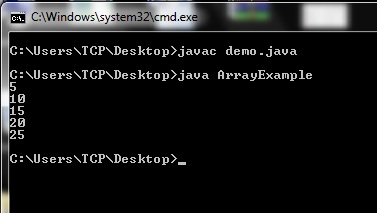# Array in Java (1D)

In this tutorial I will talk about one dimensional array in
java. In the next tutorial we will learn about multidimensional array.

## Array in Java

• Array is the collection of similar type of data having contiguous
memory location.
• Due to contiguous memory location the performance becomes
fast.
• The biggest disadvantage of array is, we can’t increase or
decrease its size after defining it.
• Array is represented by an object.
• Array is the reference variable of proxy class. The proxy
class is a class created by JVM at run time. We can get the name of the proxy
class of an array by using getClass().getName() method.
• Array reduces the program size. For example if we want to
store roll number of 100 students then we have to create 100 variables. It will
make the program very large and difficult to handle. This problem can be
solved easily by using an array of size 100.### Declare an Array in java

Array can be declared in following two ways.

Syntax

Example

In above example we simply declared a reference variable
that will store the reference of an array object.

### Create an Array in java

Array is created or instantiated using new keyword in
following way.

Syntax

Example

The above code will create an array that can store 10
values.

### Declare, Create and Initialize an Array in Java

Array can be declared, created and initialized at the same
time in the following way. In this case we don’t need to use new keyword and
specify the size.

Above code will create and array of size 5 and also initialize
it.
Array elements can be accessed using index. The indexing
starts from 0 and ends at n-1. Here n is the size of the array. The index comes
inside the square brackets []. For example if you want to access third element
of array a which we have created above then it can be done by writing a. I
have used index 2 because the first element will be at index 0, second will be
at index 1 and third element will be at index 2. In this way we can access
other elements also.

### Program for Array in Java

Let’s make a simple program that will create an array, store
some values in it and then print these values. Array elements can be accessed
one by one using loop. In below program I have used for loop, we can also use other loops. I have manually assigned
the values in an array; we can also take values from user.Explanation
In above example we have created an array of size 5 and then
initialized it. After that we are printing the values. You can see I have used
i<5, this makes the loop to run 5 times as we have 5 elements in array.
Instead of i<5, we can also use i<a.length. Actually length is a data
member of Array class which gives the size of an array.

This was all about one dimensional array in java. If you are